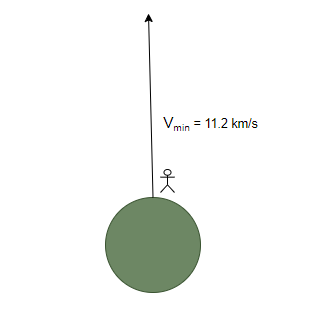# Escape Velocity

• Last Updated : 20 Oct, 2021

When a stone is thrown from the ground, it goes into the sky and falls back on the ground. Using machines, the stones can be thrown at much higher speeds. A question then comes to the mind is, with how much velocity must a person throw a stone in the sky such that it beats the gravitational pull and goes out of the earth into space. This is the idea behind the escape velocity term that is used when people talk about outer space and the missions that go for space explorations. It is essential to study the concepts behind this term to appreciate and understand how much force and effort are put into design these missions. Let’s look into these concepts in detail.

### Escape Velocity

For the objects to have velocity such that they are able to escape the gravitational pull of the earth and can go into space. One must take into account how gravity acts on the objects and does work on them. Due to this work, the kinetic energy of the object decreases and gets converted into potential energy. This decrease in kinetic energy causes the object to stop and fall back down onto the earth’s surface.

The principle of conservation of energy comes to our rescue in this case. Let’s say that the object is taken from the earth and reaches beyond its gravitational field and its velocity at that point is Vf. The energy of the object is considered to be a sum of potential and kinetic energy. Let W1 denote the gravitational potential energy of the object at infinity. Thus, the total energy of the object at infinity becomes,

E(∞) = W1 + 1/2mVf2

Let’s assume that the object was thrown with the velocity Vi from the height “h” from the surface of the earth.

The energy at this point will be,The principle of conservation of energy says that the total energy remains constant. This means that,

E(∞) = E(h + R)Since the purpose is to find the minimum velocity with which the object should be thrown to escape the earth’s gravitational pull. Let us consider that the final velocity is zero.

Vf = 0

Plugging the values of the final velocity into the equation,If the object is thrown from the ground, then h = 0,Using the relation,Using the value of “g” and “R”, the escape velocity comes out to be,

Vmin = 11.2 Km/s### Sample Problems

Question 1: Find the escape velocity for a planet whose mass is 7.35 × 1022 Kg and radius is 1.5 × 106m.

The formula for escape velocity is given by,Given: M = 7.35 × 1022 Kg

R = 1.5 × 106m

G = 6.6 × 10-11

plugging the values into the equation,⇒⇒ Vi = 7.6 × 105 m/s.

Question 2: Find the escape velocity for a planet whose mass is 14.7 × 1022 Kg and radius is 3 × 106m.

The formula for escape velocity is given by,Given: M = 14.7 × 1022 Kg

R = 3 × 106m

G = 6.6 × 10-11

plugging the values into the equation,⇒⇒ Vi = 7.6 × 105 m/s.

Question 3: Find the mass of a planet whose escape velocity is 104 m/s and radius is 2 × 105m.

The formula for escape velocity is given by,Given:  R = 2 × 105m

G = 6.6 × 10-11

Vi = 104 m/s

plugging the values into the equation,⇒⇒ 2 × 1013 = 2 × 6.6 × 10-11 × M

⇒ 0.15 × 1024 = M

⇒ 1.5 × 1023 = M

Question 4: Find the mass of a planet whose escape velocity is 2 × 104 m/s and radius is 2 × 105m.

The formula for escape velocity is given by,Given:  R = 2 × 105m

G = 6.6 × 10-11

Vi = 2 × 104 m/s

plugging the values into the equation,⇒⇒ 8 × 1013 = 2 × 6.6 × 10-11 × M

⇒ 4 × 0.15 × 1024 = M

⇒ 6 × 1023 Kg = M

Question 5: Find the escape velocity if the radius of the earth is increased by 4 times.

The formula for escape velocity is given by,In earth’s case it becomes,Notice that,The radius is made four times, this means that the velocity must be doubled.

Escape Velocity of earth is 11.2 Km/s.

Escape Velocity of earth with enlarged radius will be 22.4 Km/s.

My Personal Notes arrow_drop_up